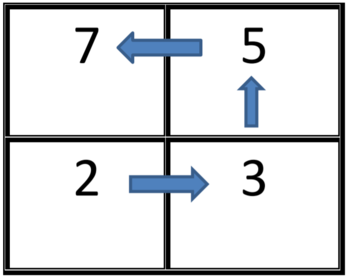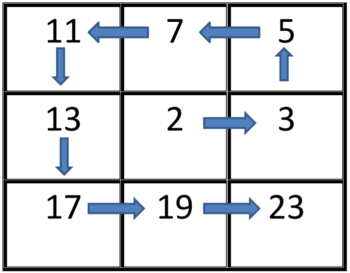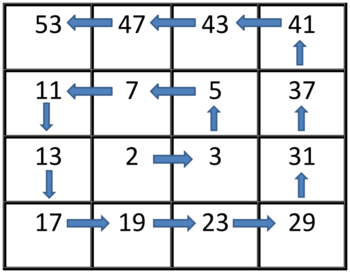# Prime SpiralPKP007National High School Prog...
Limits 1s, 512 MB

You have to make a 2-D spiral square $N \times N$ grid with prime numbers. Sounds complicated? Well, it's always easier to understand by visual representation.

For $N = 2$, the grid will be:For $N = 3$, the grid will be:For $N = 4$, the grid will be:and so on...

In this problem, you will be given the value of $N$ and $Q$, number of queries. For each query, you will be given the index of a cell $(X, Y)$. The cells are 1-indexed where $X$ denotes the row number and $Y$ denotes the column number. You have to find out the prime number in that cell. For example: if $N = 2$ and cell number is $(2, 1)$, then the prime number is $2$, Again, if $N = 3$ and cell number is $(3, 2)$, then the prime number is $19$.

## Input

First line of input will contain two integers $N$ and $Q$. $N$ denotes size of the $N \times N$ square grid and $Q$ denotes the number of queries.

Each of the next $Q$ lines will contain two positive integers $X$ and $Y (1 \leq X, Y \leq N)$.
Here, $(X, Y)$ denotes the cell number.

$1 \leq N \leq 10$
$1 \leq Q \leq 100$

$1 \leq N \leq 100$
$1 \leq Q \leq 10^4$

$1 \leq N \leq 1000$
$1 \leq Q \leq 10^5$

## Output

For each query, print the prime number of that cell in the grid.

## Samples

InputOutput
3 2
1 3
2 1

5
13

InputOutput
4 3
1 2
4 1
4 4

47
17
29


### Notes

A prime number is a natural number greater than 1 that is divisible only by itself and 1. For example, 2, 3, 5, 7, 11, etc.

### Submit